# Introduction to Coordinate Reference System

The coordinate reference system (CRS) is one of the most important functions of GIS. It is a coordinate-based local, regional or global system used to locate geographical features that occupy a position in space, and it allows users to analyse geographic data using accurate locations and measurements.

(Coordinate reference systems and spatial reference system (SRS) are synonyms and are commonly interchanged.)

The two types of coordinate reference system in GIS are:

For example in ArcMap, when a spatial data is created or added, the coordinate or spatial references may need to be set.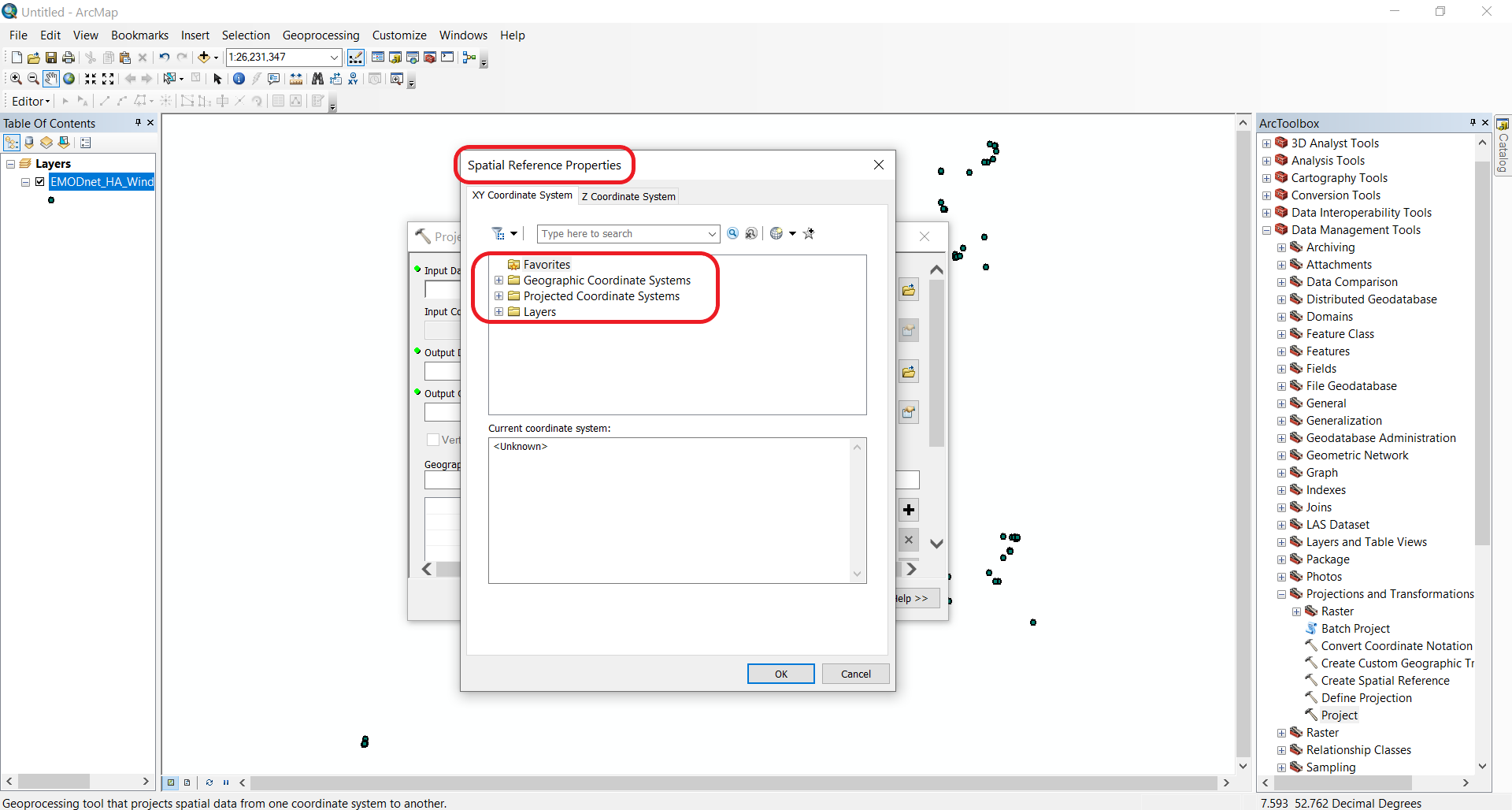Spatial reference properties window in ArcMap

Both geographic coordinate and projected coordinate systems will come with many options.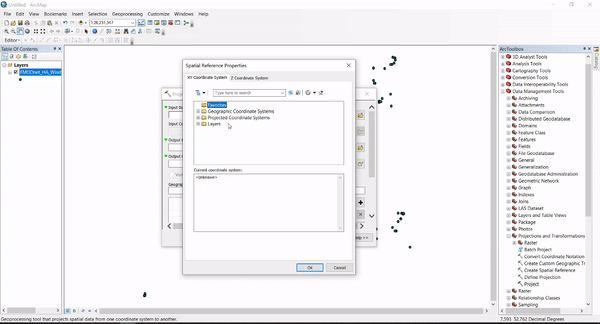Coordinate reference systems in ArcMap

So let’s discuss what these are separately.

## Geographic coordinate system

Geographic coordinate system is used to define locations on the three-dimensional Earth. GCS shows where a certain location is on the surface of the Earth.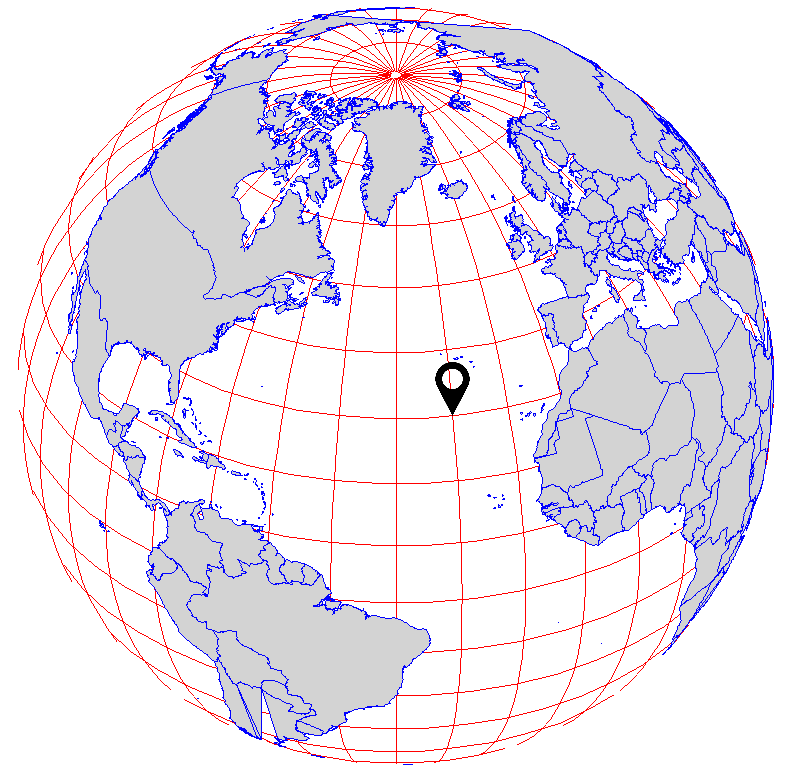Geographic coordinate system definew locations on the three-dimensional Earth

Geographic coordinate system is defined by:

### Geodesy

Geodesy is the science associated with the measurement and portrayal of the Earth. Geodesy is used to determine the size and shape of the Earth, as well its features (e.g. mountains) and elements (e.g. terrestrial gravity and tides).

#### Ellipsoid

Geodesists build simple mathematical models to be able to capture the shape of the Earth and its features. For this, they have adopted the ellipsoid (or spheroid) as the most basic model. This is also referred to as geometric model.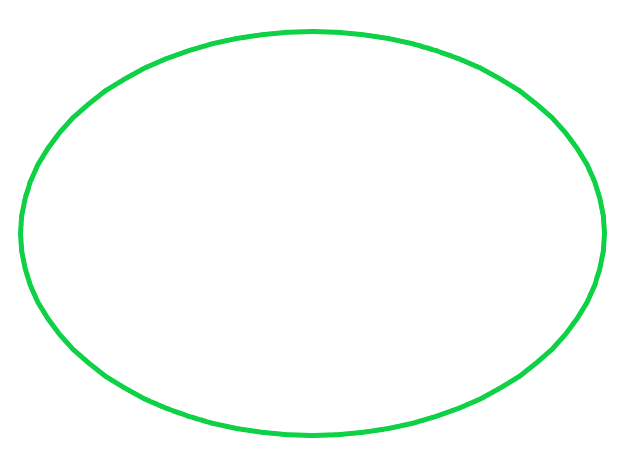Ellipsoid

The ellipsoid is an idealised representation of the physical Earth, with a completely smooth, uninterrupted surface.

Note

Since the Earth is spinning around its axis, there is a slight flattening at the poles and a slight bulging at the equator, hence the ellipsoid is used as a model.

The ellipsoid has a longer semi-major axis (a) and a shorter semi-minor axis (b). The difference between the ellipsoid and the sphere is measured by its flattening (f = (a-b)/a), or the reduction in the minor axis relative to the major axis.

The circumference of the earth is about 1/300th smaller around the poles. This translates to the Earth being around 44km wider than it is tall.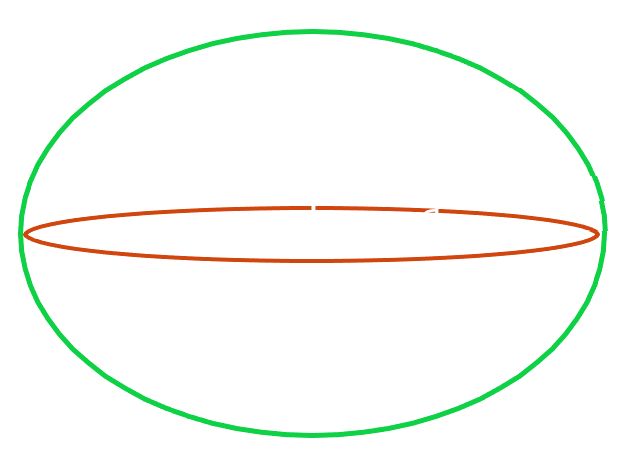Ellipsoid with the semi-major (a) and semi-minor axis (b)

In addition to the flattening and bulging, the different topological features are also account for Earth’s irregular, lumpy shape.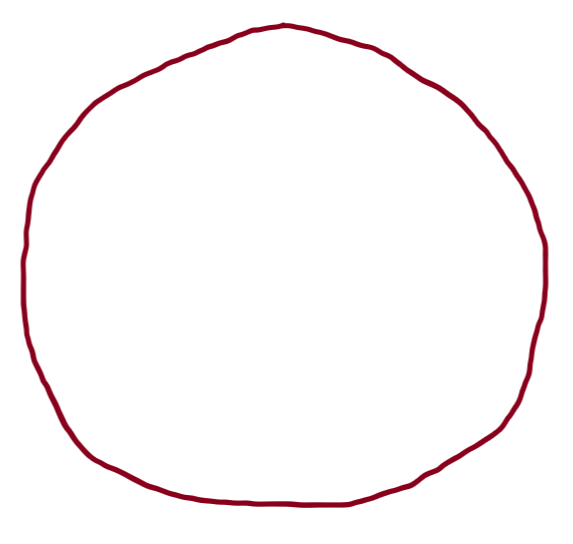Irregular shape of the Earth (simplified illustration)

So let’s imagine the modeling process using the ellipsoid.

The ellipsoid placed on top of this lumpy shape won’t be able to perfectly model the surface all around. It will only “fit” with the surface at a particular area. This is why the ellipsoid can only be applied at one part of the Earth at the time.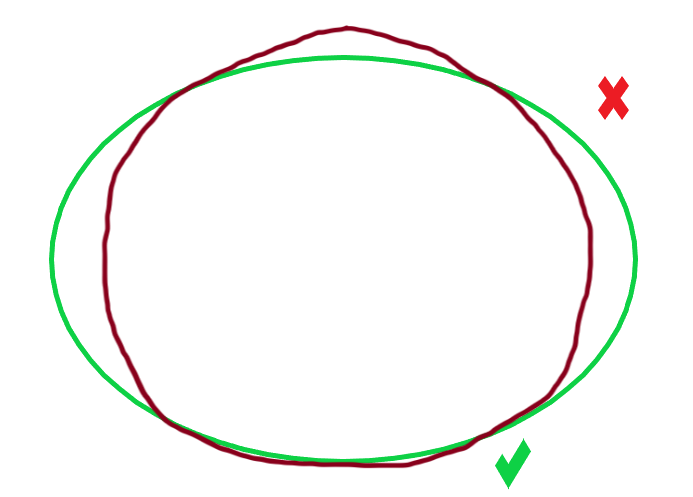Ellipsoid used to model the Earth (simplified illustration)

To be able to specify at which part of the planet the ellipsoid is applied to, datums are used.

#### Datum

Horizontal datums model positions on the surface of the Earth using latitude (y) and longitude (x), while vertical datums are used to model elevations (z) accounting for terrain, bathymetry, water level and man-made structures.

##### Horizontal datum

A horizontal datum uses a reference ellipsoid that is “pinned” to the Earth at a fixed surface location. This reference location is called marker or benchmark.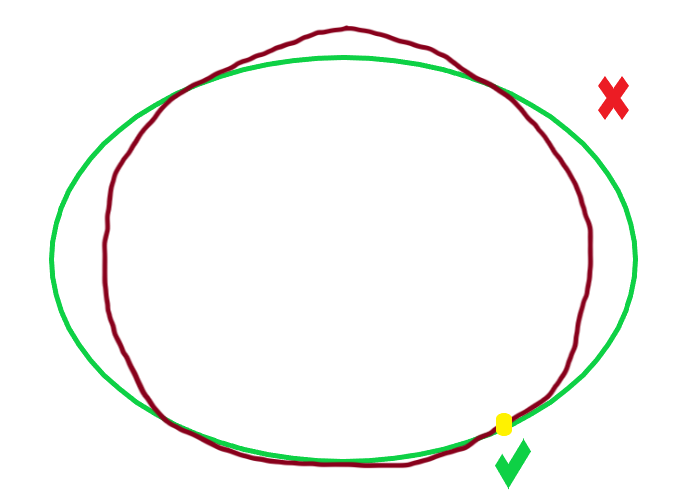Ellipsoid with a marker (simplified illustration)
and a marker location in Kansas, USA (Source: Wikipedia)

This marker is then used to assign angular units of longitude and latitude to locations at that particular area of the Earth.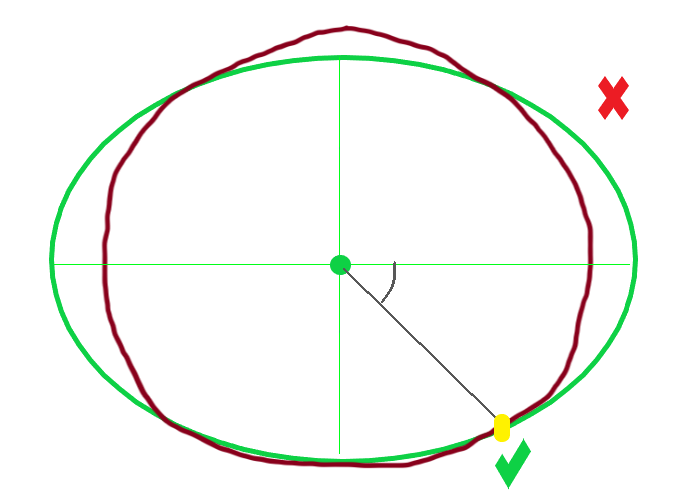Datum (simplified illustration)

There are different datums designed for different parts of the world. In fact, almost every country has its own datum (local datum). For example, the datum NAD83 (North American Datum 1983) is used to model the North American continent and the NZGD2000 (New Zealand Geodetic Datum 2000) is used for New Zealand and its offshore islands.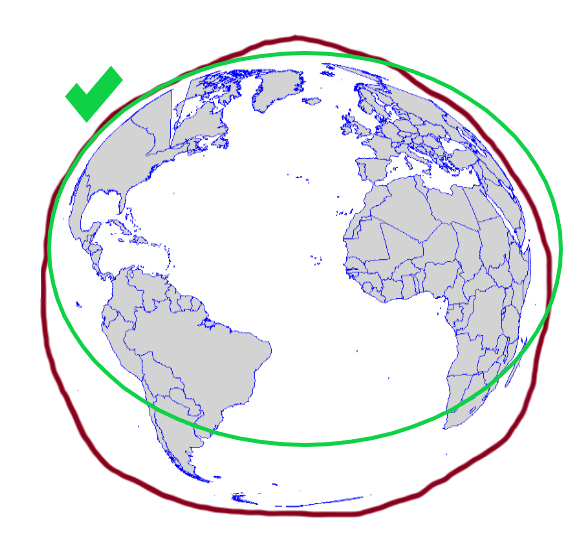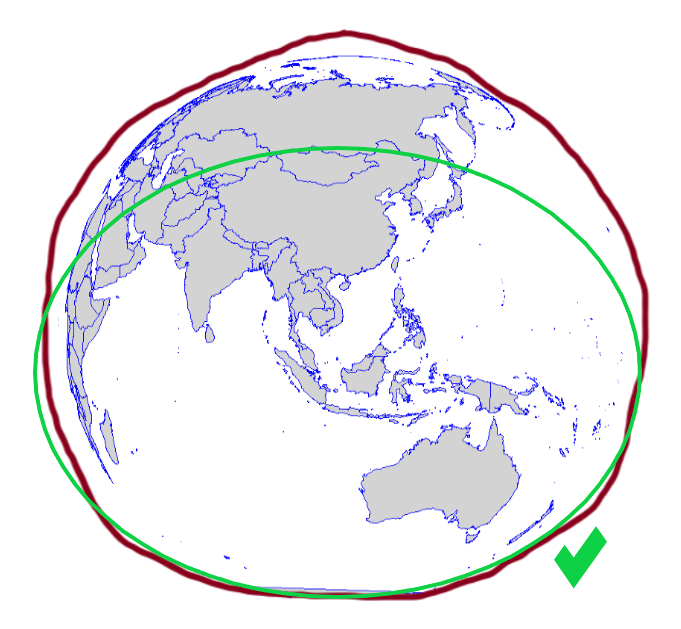The ellipsoid known as WGS84 or World Geodetic System 84 is the most widely used and well-known datum.

WGS84 is global datum format. It uses the centre of the Earth to connect the ellipsoid and provides a generalised model for the whole of the planet.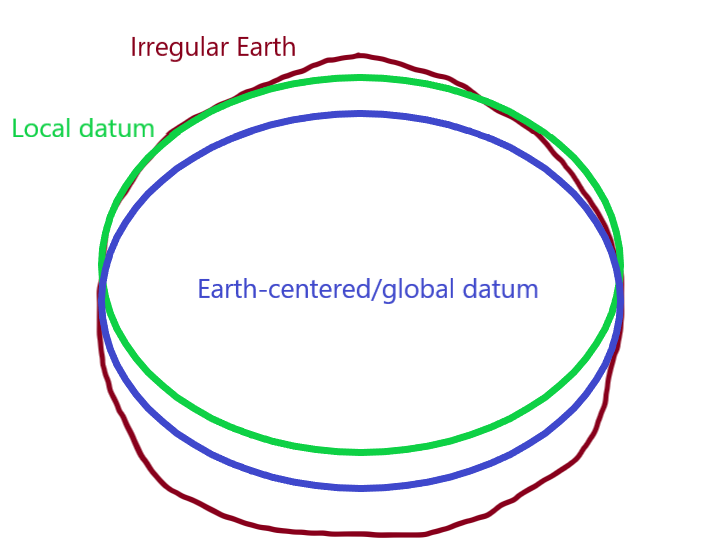Local and global datum (WGS 84) (simplified illustration)

##### Vertical datum

As previously discussed, the shape of the Earth is not perfectly round and its surface is quite uneven: there are different topographic features (e.g. mountains, valleys, trenches). This uneven surface has an effect on Earth’s gravity: higher density rocks will pull water creating a bulge, while water is drawn away from lower density rocks. Thus, gravity is different at different parts of the Earth.

To overcome these gravitational differences, geodesists use the geoid to measure surface elevation. The geoid is also known as gravity-related model.

This 3D model shows the variations in Earth’s gravity and the elevation differences
(Source: Geoid viewer)
Use the tools on the left to interact with the model

Removing the effects of the winds and tides, and in fact land masses that cause gravitational differences, the geoid takes the global mean sea level as a base (as if the sea would uniformly cover the planet) to measure surface elevations.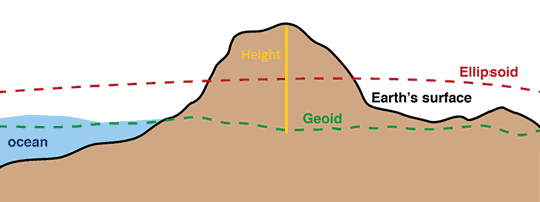Contrast of the geoid model with an ellipsoid (modified) (Source: USGS)

At a certain location, vertical height will be given in reference to the geoid.

The above mentioned WGS84 is used by Global Positioning System (GPS) satellites and can be used globally to obtain latitude, longitude and elevation coordinates at any location. However, at specific areas local datums can still provide better coverage than WGS84. For example, OSGB36 (Ordnance Survey National Grid reference system) is a better approximation to the geoid covering the British Isles than the global WGS84.

Note

Maps made using different datums will give different coordinates for the same position. This is called ‘datum shift’.

The GPS consists of a system of 24 satellites, each orbiting the Earth every 12 hours on distinct orbits at a height of 20,200km and transmitting radio pulses at precisely timed intervals. To determine position, a receiver must make exact calculations from the signals, the known positions of the satellites and the velocity of light. Positioning in three dimensions (y,x,z) requires that at least four satellites are above the horizon and accuracy depends on the number of such satellites and their positions.

GPS is very useful for locating objects that move and for direct capture of the location of fixed objects.

Satellite map (Source: Esri)
Zoom in and use the tools on the left to interact with the map

### Angular units

GCS use angular units to describe locations on the Earth’s surface. (Remember, this is based on the ellipsoid and the geoid.) These are the latitude (φ) and longitude (λ) displayed on a spherical coordinate system and are measured in degrees.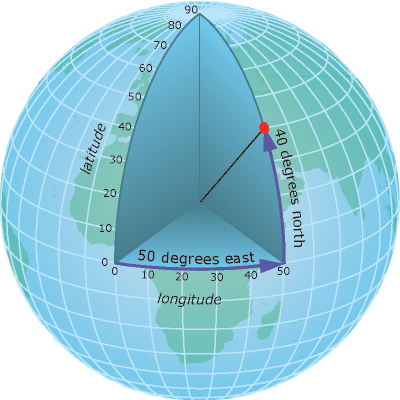Latitude and longitude on an elipsoid (Source: Esri)

Latitude lines run parallel to the equator and divide the Earth into 180 equally spaced sections from north to south.Latitude lines running from east to west

Longitude lines run perpendicular to the equator and converge at the poles. Longitudes are measured from zero to 180 degrees east or west of the prime meridian (0 degrees running through Greenwich, UK).Longitude or meridian lines running from north to south

This network of lines representing meridians and parallels are also known as graticules.Graticular network

The classic representation of latitude and longitude coordinates is in degrees, minutes and seconds (DMS).

The example below shows London on the map with the coordinates displayed in degrees, minutes and seconds.

London

The degrees, minutes and seconds format uses the sexagesimal (base 60) system which is difficult to use in the digital world. Instead, the decimal degrees format (base 10 system) is used. So let’s see how to convert degrees, minutes and seconds to decimal degrees, and decimal degrees to degrees, minutes and seconds.

DMS to DD

Location DMS (degrees, minutes and seconds) DD (decimal degrees)
London, UK 51° 30’ 35.5140’’ N, 0° 7’ 5.1312’’ W ???

Leaving the degree value untouched, divide the minute with 60 and the second with 3600. Then, add them up (degree + minute + second). The final value will be the decimal degrees format.

Let’s take the latitude and latitude values separately from the above example.

Latitude: 51 degrees 30 minutes 35.5140 seconds

D (51 degrees) + M (30/60) + S (35.5140/3600) = 51.509865°

Longitude: 0 degrees 7 minutes 5.1312 seconds

D (0 degrees) + M (7/60) + S (5.1312/3600) = 0.118092°

Location DMS (degrees, minutes and seconds) DD (decimal degrees)
London, UK 51° 30’ 35.5140’’ N, 0° 7’ 5.1312’’ W 51.509865°, 0.11809°

DD to DMS

Here, let’s take the latitude example only.

Latitude: 51.509865

1, The degree value remains the same:

Degrees = 51

2, The decimal value gets multiplied by 60 and the minute will be the number before the decimal separator:

0.509865x60 = 30.5919

Minutes = 30

3, The remaining number after the decimal separator gets multiplied by 60 as well. This will give the second.

0.5919x60 = 35.514

Seconds = 35.514

51° 30’ 35.514’’

When using DMS and DD, directions are specified by using N, E, S and W or +/-, respectively (i.e. y above the equator and x east of the prime meridian are positive, and y below the equator and x west of the prime meridian are negative).

Apart from DMS and DD, there is also a third format to display locations. This is called degrees decimal minutes (DDM).

Degrees, minutes and seconds can also be converted to degrees decimal minutes.

DMS to DDM

Using the same example, starting from the back:

Latitude: 51 degrees 30 minutes 35.5140 seconds

1, Divide the seconds by 60:

35.5140/60 = 0.5919

30 + 0.5919 = 30.5919

3, The degree value remains the same:

51°30.5919’

Longitude: 0 degrees 7 minutes 5.1312 seconds

7.08552’

Location DMS (degrees, minutes and seconds) DD (decimal degrees) DDM (degrees decimal minutes)
London, UK 51° 30’ 35.5140’’ N, 0° 7’ 5.1312’’ W 51.509865°, 0.11809° 51°30.5919’, 0°7.08552’

Tip

The website PGC Coordinate Converter is useful to convert all three formats.

Batch Convert Tool allows to convert UK Grid Reference, easting and northing, and decimal degrees formats.

Google Earth Pro also gives the option to display and convert all formats (Tools –> Options).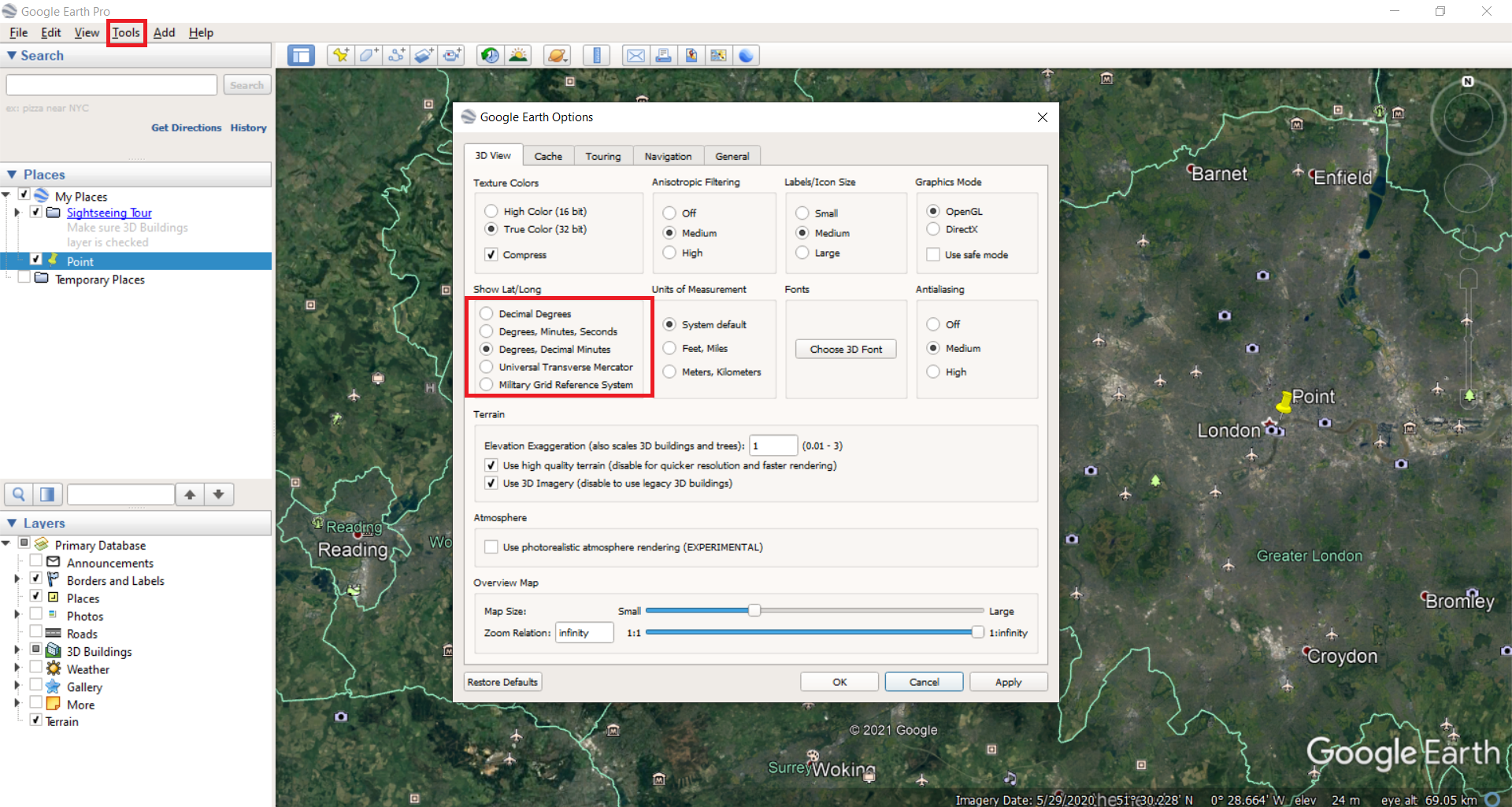#### Distance

As mentioned before, latitude and longitude are applied to the three-dimensional Earth to describe location. However, they are not very useful to measure linear distances. This is because of the oblate spheroid shape of the Earth.

Latitude lines are concentric circles that become smaller near the poles while the distance between them remains roughly the same. (The distance between two degrees is slightly bigger at the poles than at the equator because of the flattening. The average distance between two latitude degrees is 111km. One minute of latitude is 1.86km which equals one nautical mile.)

Latitude Poles 80° N/S 40° N/S Equator
Distance between two degrees 111.69km 111.66km 111.03km 110.57kmLatitude lines, the thicker lines mark the degrees at 40° N/S and at the equator

Longitude lines converge at the poles and thus the distance between two meridians is different at every parallel.

Longitude Poles 80° N/S 40° N/S Equator
Distance between two degrees - 19.39km 85.39km 111.32kmLongitude lines, green parallels mark the degrees at 40° N/S and at the equator

The equator is the only place where the linear distance corresponding to one degree latitude is approximately equal the distance corresponding to one degree longitude. (Note that the illustration below shows the latitude and longitude lines at every 10° for easier visualisation.)Distances are almost equal between the latitudes and longitudes at the equator

Note

Formula to calculate distances at one minute latitude and at one second latitude at the equator:

1°= 110.57km

1’ = 110.57/60 = 1.84283km

1’’ = 1.84283/60 = 30.7138m

The same formula applies at one degree/minute/second at all latitude and longitude lines, except at the poles where the longitude lines converge.

Interesting fact

##### Measuring distance on the sky

When holding out one hand at arm’s length with one eye closed:

• The little finger at an arms length is about 1° wide.

• Three middle fingers spans about 5°.

• The width of a fist is approximately 10°.

• The tip-to-tip span between the index and little finger is 15°.

• The tip-to-tip span between the thumb and little finger is 25°.A handy way to measure distances in the sky (Source: timeanddate.com)

Note

• 24 hours equals with Earth’s full rotation around its axis (360°).

• In one hour, Earth turns 15°.

• In every four minutes, Earth turns one degree.

#### Direction

Maps tend to show a straight line as a shortest distance between two points.

However, on spherical Earth this is not the case. Instead, this is better illustrated with an arced line, called great circle, that shows the shortest distance between two points on a sphere.

Long-distance air traffic uses great circle routes routinely, plotting great circles to fly the shortest distance between two locations.

Flight path between London and Tokyo

The video below explains and visualises the concept of great circles and straight lines really well.

Great Circles are Straight Lines - video

This issue of distance and direction at different latitude and longitude is bridged and accounted for by most GIS software and discussed in the projected coordinate system section below.

## Projected coordinate system

Whilst GCS shows where a certain location is on the Earth’s surface, the projected coordinate system (PCS) shows how the three-dimensional Earth should be projected on a two-dimensional flat surface to accurately display that same location.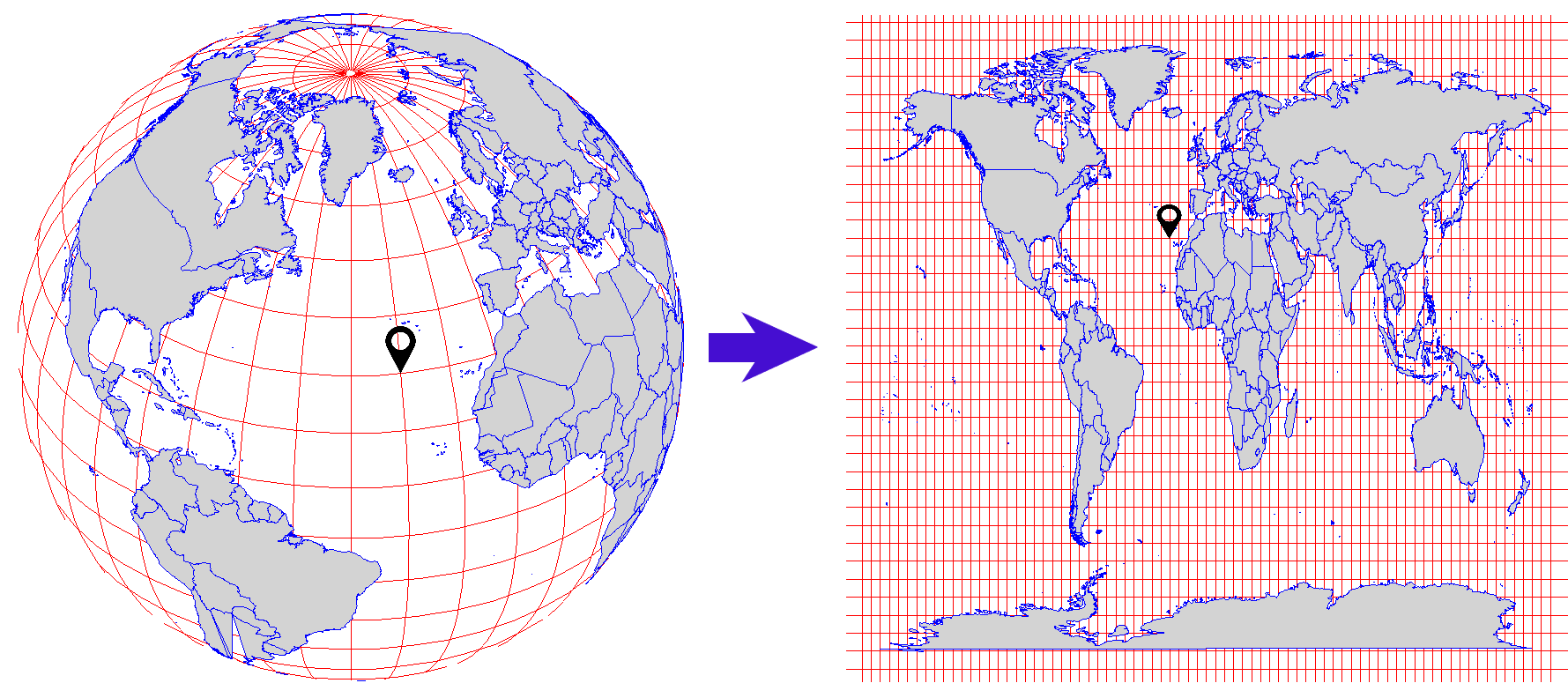Projected coordinate system

### Map projection

The transformation used to convert the three-dimensional spherical Earth onto a two-dimensional flat surface is referred to as a map projection. A map projection uses mathematical formulas to relate spherical coordinates on the globe to planar coordinates using the Cartesian coordinate system.

#### Cartesian coordinate systemCartesian coordinate system

The Cartesian coordinate system assigns two coordinates to every point on a flat surface by measuring distances from an origin parallel to two axes drawn at right angles:

• Horizontal (X / Easting) axis runs from east to west, ranging from +180° to -180°.

• Vertical (y / Northing) axis runs from north to south, ranging from +90° to -90°.

The point at which the axes intersect is called the origin (0,0). Locations are defined relative to the origin, using the notation (x,y).

When working with data in a geographic coordinate system, longitude values are equated with the X axis and the latitude values are equated with the Y axis.

#### Projection types

As it was discussed in the Introduction to Cartography chapter, during the map projection process distortion occurs affecting shape, area, distance and direction. Depending on the on the map’s content and purpose, different projection types and methods are used to minimalise distortion.

The three projection types are:

• Cylindrical (normal, transverse, oblique)

These projections are used for the entire earth and for areas at the equator.

• Conical (secant, tangent)

These projections are used for the mid-latitudes.

• Planar/Azimuthal (Gnomonic, Stereographic, Orthographic)

These projections are used for the poles.Projection types (Source: Wikipedia)

#### Projection methods

Within the projection types, there are different kind of methods used. The most commons are:

##### Lambert Conformal Conic

Lambert Conformal Conic is used for aeronautical charts, portions of the State Plane Coordinate System, and many national and regional mapping systems. It is also one of the best projection methods for middle latitudes.Lambert Conformal Conic projection
##### Azimuthal Equal Area

This projection maintains land features at their true relative sizes while simultaneously maintaining a true sense of direction from the center. It is best suited for individual land masses that are symmetrically proportioned, either round or square.Azimuthal Equal Area
##### Equidistant Cylindrical (Plate Carrée)

This projection is neither equal-area nor conformal. One of the simplest of all map projections and one of the oldest.Equidistant Cylindrical projection

There are also projection methods that use their own coordinate system.

##### Universal Transverse Mercator (UTM)

UTM is based on the Mercator projection, but in the transverse rather than equatorial aspect: like wrapping a cylinder around the poles rather than around the equator.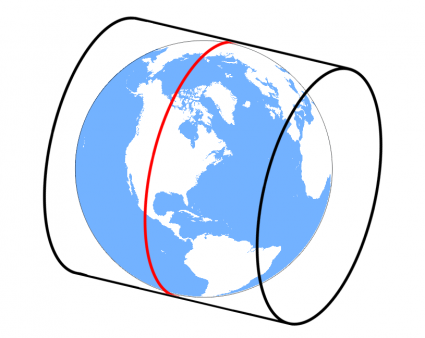Transverse Mercator projection (Source: GISGeography)

UTM divides the world into 60 grids that are all 6 degrees wide in longitude.

Each grid has a central meridian of 500,000m and each is segmented into 20 latitude bands that are 8 degrees high.

Along the latitude lines, each grid lettered starting from C at 80°S, increasing until X (English alphabet), omitting the letters I and O. The last latitude band, X is extended an extra 4 degrees ending at 84°N latitude, thus covering the northernmost land on Earth. Latitude bands A and B, and Y and Z bands cover the western and eastern sides of the Antarctic and Arctic regions respectively.

Each grid is also divided into north (N) and south (S) depending on its location from the equator.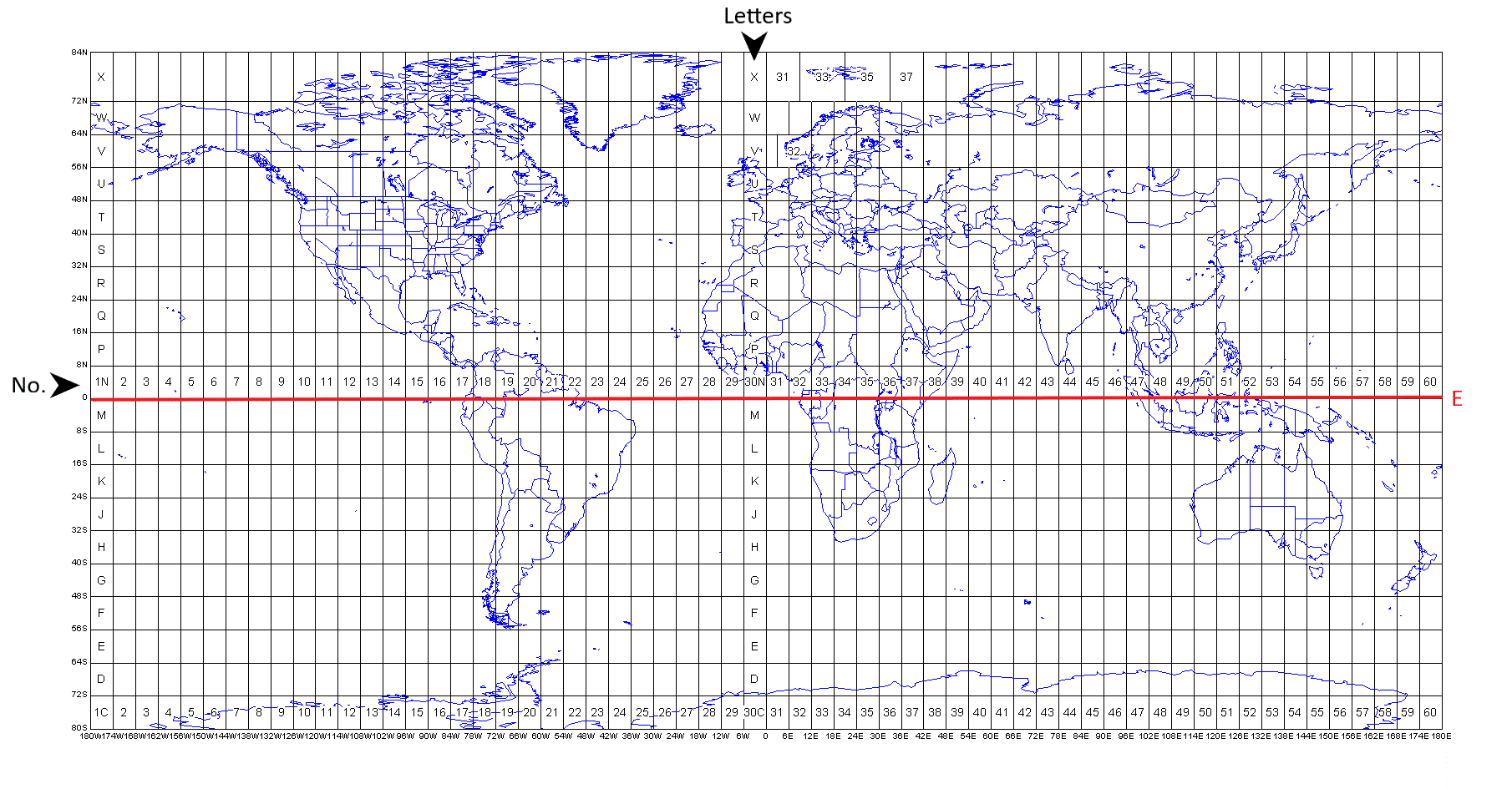UTM zones (Source: modified after dmap.co.uk)